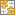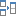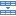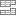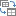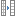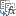# How to calculate percentage variance in Excel - percent change formula

This short tutorial explains how to make an Excel formula for variance between two cells.

In Microsoft Excel, there are 6 different functions for calculating variance. However, none of those functions is fit to find percentage variance between two cells. The reason is the inbuilt functions such as VAR, VAR.S, VAR.P, etc. are designed to find variance in the classical sense, i.e. how far a set of values are spread out from their average. A percent variance is something different. In this article, you will find the right Excel variance percentage formula.

## What is the percentage variance?

A percentage variance, aka percent change, describes a proportional change between two numbers, an original value and a new value.

The percent variance formula shows how much something changes between two periods. For example, you can calculate variance between sales in this year and last year, between a forecast and observed temperature, between a budgeted cost and the real one.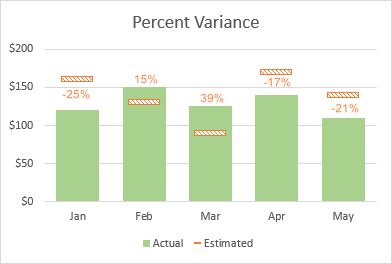## How to calculate variance percentage in Excel

There are two basic formulas to find percent variance in Excel.

### Formula 1

It is the classic percent change formula.

(new_value - old_value) / old_value

### Formula 2

A simplified Excel variance percentage formula that returns the same result.

new_value / old_value - 1

As an example, let's find a percent variance between the estimated sales in B2 and actual sales in C2. Assuming the estimated number is the "baseline" value and actual is a "new" value, the formulas take this shape:

Formula 1:

`=(C2-B2)/B2`

Formula 2:

`=C2/B2-1`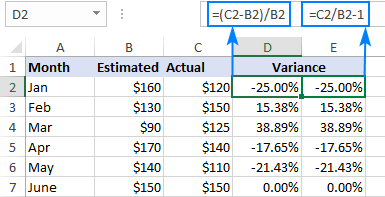By default, the results are displayed as decimal numbers. To show percentages, apply the Percentage number format to the formula cells. For this, click the Percent Style button in the Number group on the Home tab or press the Percentage format shortcut Ctrl + Shift + %.

### How Excel percent variance formula works

If you were to find percent change manually, you would take an old (original) value and a new value, find the difference between them and divide it by the original value. To get the result as percentage, you would multiply it by 100.

For example, if the original value is 160 and the new value is 120, the percent variance can be calculated in this way:

`=(120-160)/160`

`=-40/160`

`=-0.25`

`-0.25*100 = -0.25%`

When you apply the Percentage number format in Excel, a decimal number is displayed as a percentage automatically, therefore you do not need to multiply by 100.

### Prevent #DIV/0 errors

If your data set contains some zero values, wrap the formulas in the IFERROR function to prevent division by zero errors (#DIV/0!).

`=IFERROR((C2-B2)/B2, 0)`

`=IFERROR(C2/B2-1, 0)`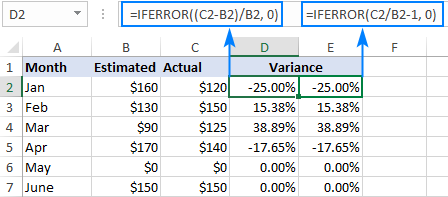### Calculate discount percentage

The percent change formulas come in handy to work out a discount percentage. So, ladies, when you go shopping, remember this:

`discount % = (discounted price - regular price) / regular price`

`discount % = discounted price / regular price - 1`

The formulas display a discount percent as a negative value because the new discounted price is smaller than the old regular price. To output the result as a positive number, wrap the formulas into the ABS function. For example:

`=ABS((C2-B2)/B2)`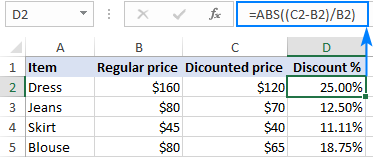## How to calculate percent variance for negative numbers

If some of the original values are represented by negative numbers, the above formulas won't work. A common workaround is to make the denominator in the first formula positive. For this, use the ABS function:

(new_value - old_value) / ABS(old_value)

With the old value in B2 and the new value in C2, the formula goes as follows:

`=(C2-B2)/ABS(B2)`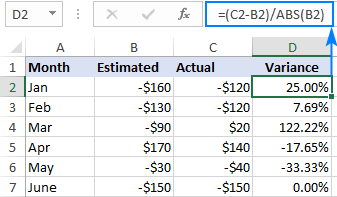Note. Although this ABS adjustment is technically correct, the formula may produce misleading results in case the original value is negative and new value is positive, and vice versa.

As you see, calculating percent change in Excel is very easy. For hands-on experience, you are welcome to download our sample workbook with all the Variance Percentage Formulas discussed in this tutorial. I thank you for reading and hope to see you on our blog next week!

### 7 responses to "How to calculate percentage variance in Excel - percent change formula"

1.sameer says:

Nice

2.Mohamed Ali says:

Hello,
I'm calculating a variance of two points. Satisfaction rate of students in Q3 vs Q4. 88.1% for Q3 and 92.3% for Q4. Is it appropriate to use percent variance or straight variance no percent here? Please advise.

Thanks,

3.Tony says:

Thank you. Great!

4.Ahsum says:

Formula for calculating year-on-year % variations:
IF(ISERROR((D4/C4)-1);"n.a.";IF(((D4/C4)-1)>1;">100.0%";IF(((D4/C4)-1)<-1;"<-100.0%";((D4/C4)-1))))

5.Philip Assampong says:

How do I do V look up

6.Ralf says:

Hello Svetlana,
Good article, thank you!
I have a follow up question:
How would you show the WoW (week over week) variance without changing the formula to point to different columns every week?
Say I have week 1 to week 52 columns, and in column 53 I show WoW. For example, this WoW column would point to week 25 vs week 24 to show the variance of week 25 to week 24. The week after however, it needs to point to week 26 vs week 25; and the week after that to week 27 vs week 26; and so on.
What's the solution to using a fixed WoW formula that needs no change every week?
Thank you,
Ralf

Ultimate Suite for Microsoft Excel